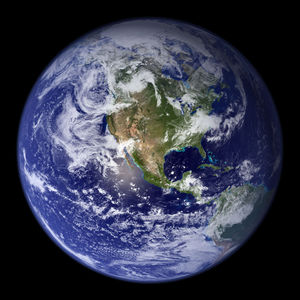# Gravitational fieldFigure 1. The Earth, and every other massive object, has a gravitational field that causes other massive objects to interact with it.

A gravitational field is a field induced by any object with mass, which will interact with other massive objects by applying a force on it. Gravitational fields are expressed in Newtons per kilogram (N/kg), which is the same unit as acceleration. This means that any massive object present in another's gravitational field will accelerate towards it, and vice versa. The field of an object is given by the equation:

where:

Using the equation above provides the value for gravitational field strength. Specifically, using the mass and radius of the Earth, the equation shows that the acceleration due to gravity near the surface of the Earth is: (this value changes the further or closer an object is relative to the centre of the Earth).

This value means that all objects will fall at the same rate near the surface of the Earth (ignoring air drag and minor changes due to altitude and latitude).

Since the gravitational field of the Earth is conservative it must have a potential energy associated with it. This means that any object at a certain height from the surface of the Earth has a potential energy, which can be translated into kinetic energy as the object falls. Humans can make use of falling objects such as water to transform this potential energy into something useful, like electricity. Hydroelectric facilities make use of the moving water in a river in order to create electricity, which depends on this potential energy in order to operate.